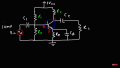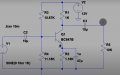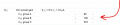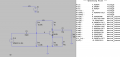# common emmitter bjt amplifier question

#### yef smith

Joined Aug 2, 2020
488
Hello,in the circuit bellow i have a bjt circuit
where C_B is for the AC signl to bypass R_E
R1 and R2 are voltage divider DC bias.
C1 and C2
Vin is the small signal voltage.
How do i choose the values of C2 C1 and Cb?

there is an implementation bellow but there is no explanation regarding why they chose each paramter.Last edited:

#### ericgibbs

Joined Jan 29, 2010
17,416
How do i choose the values of C2 C1 and Cb?
Hi yef,
You start by determining the required bandwidth specification, gain, in/out impedances.
IE: the specification of the amp.

E

Edit: post your LTS asc file.

Last edited:

#### Ian0

Joined Aug 7, 2020
7,441
It's the usual equation f=1/(2πRC) where f is the cutoff frequency.
All you have to do is to identify which R is relevant to the equation.
With C1, R is R1 and R2 in parallel
with Cb it is Re
with C2 it is Rl (although I am assuming that you have made a sensible choice for Rl and Rc, and that Rl is at least ten times the value of Rc.
Another thing to note is that there will be a 3dB reduction in gain at your cutoff frequency for EACH RC circuit, so the overall response will be 9dB down at the frequency you choose.
To make things more predictable choose one of the three time constants to be the one that sets the frequency response, then set the other two a decade lower so that they don't have much effect.

#### LvW

Joined Jun 13, 2013
1,644
With C1, R is R1 and R2 in parallel
with Cb it is Re
with C2 it is Rl (although I am assuming that you have made a sensible choice for Rl and Rc, and that Rl is at least ten times the value of Rc.
I think, the above information about the time constants (relevant resistors) is not correct.
In the following, I am using the notation as given in post#1.

* With C1: We have three resistors in parallel: R1||R2||r_in (r_in is the dynamic input resistance at the base node, lower kOhm range for RE bypassed with C3)
* With C3: Two parallel resistors RE||r_e (r_e is the dynamic input resistance at the emitter with r_e=1/gm, a pretty low value dependinmg on the DC qiescent current Ie)
* With C2: Three parallel resistors: RL||Rc|| r_out (r_out is the output resistance at the collector node, larger kOhm range - can often be neglected against Rc||RL).

Obviously, all three capacitors will cause a highpass response.
The dominating capacitor will be the one with the smallest time constant (greatest cut-off frequency):
In this case, capacitor C3 in parallel to RE because Te=C3*(RE||r_e) with r_e=1/gm (Example: Ie=1mA; r_e=26mV/1mA=26 Ohm)

Last edited:

#### yef smith

Joined Aug 2, 2020
488
Hello, what i posted was someone else design i want to understand.
i am starting with the datasheet shown bellow.

https://pdf1.alldatasheet.com/datasheet-pdf/view/558562/PHILIPS/BC847B.html
the LTspice schematic file npb_ce.asc is attached.
the cuurent bias point is shown bellow.
I want to change the biasing so Vce=5V and Ic=10uA so my beta(Hfe) will be 150.
What is the proper methmatical approach for my circuit to change the bias point to what i want?
Thanks.#### Attachments

• 1.6 KB Views: 1

#### yef smith

Joined Aug 2, 2020
488
Hello , its not an error i have copied the example bellow.
What is the math for changing the bias point of this circuit to Vce=5V and Ic=10uA?
Thanks.

#### ericgibbs

Joined Jan 29, 2010
17,416
hi yef,
What is the math for changing the bias point of this circuit to Vce=5V and Ic=10uA?

Surely you do not mean 10uA for Ic.???

E

#### yef smith

Joined Aug 2, 2020
488